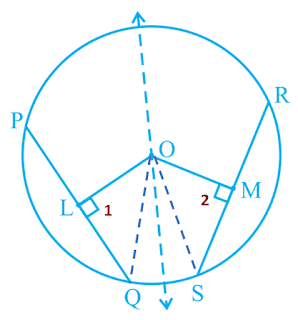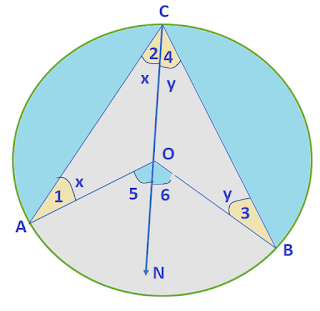### Resource Centre Mathematics

Resource Centre Mathematics Mathematics worksheet, mathematics basic points and formulas, mathematics lesson plan, mathematics multiple choice questions Workplace Dashboard CBSE Syllabus For Session 2023-24 For  :   Classes IX & X    |   Classes XI & XII Watch Videos on Maths Solutions CLASS IX MATHEMATICS FORMULAS &  BASIC CONCEPTS

# CHAPTER - 10 CLASS - IX

Explanation of all theorems on Circle and cyclic quadrilateral, chapter 10, class IX, Theorem 10.1, 10.2, 10.3, 10.4, 10.5, 10.6, 10.7, 10.8, 10.9, 10.10, 10.11, 10.12.

## Theorem 10.1 : Equal chords of a circle subtend equal angles at the centre.

Given: A circle C(O, r) in which chord AB = Chord CD  or  AB = CD

To Prove: AOB = COD

Proof: In AOB and COD

OA = OC ..... ( Equal radii)

OB = OD ..... ( Equal radii)

AB = CD ..... ( Given)

By SSS rule,  AOB  COD

⇒ AOB = COD ..... (By CPCT)

*************************************************

## Theorem 10.2 : If the angles subtended by the chords of a circle at the centre are equal, then the chords are equal. (Converse of Theorem 10.1)

Given: A circle C(O, r) in which ∠AOB = ∠COD

To Prove: AB = CD

Proof: In △AOB and △COD

OA = OC ..... (∵ Equal radii)

OB = OD ..... (∵ Equal radii)

∠AOB = ∠COD ..... (∵ Given)

∴ △AOB ≌ △COD ..... By SSS ≌ rule

⇒ ∠AOB = ∠COD ..... (By CPCT)

*************************************************

## Theorem 10.3 : The perpendicular from the centre of a circle to a chord bisects the chord.

Given: A circle C(O, r) in which OM⊥ Chord AB
or  OM ⊥ AB

To Prove: Point M bisects Chord AB

or AM = BM

Construction: Join OA and OB

Proof:  In AOM and △BOM

∠AMO = ∠BMO ........ (∵ Each = 90)

OA = OB .......  (∵ Equal radii)

OM = OM ..... (∵ Common Side)

∴ AOM  BOM ...... By RHS ≌ rule

⇒ AM = BM ..... (By CPCT)

*************************************************

## Theorem 10.4 : The line drawn through the centre of a circle to bisect a chord is perpendicular to the chord.

Given:  A circle C(O, r) in which M is the mid point of the chord AB  Or  AM = BM

To Prove:  OM ⊥ AB

Construction:  Join OA and OB

Proof:  In AOM and △BOM

AM = BM ........ (∵ Given )

OA = OB .......  (∵ Equal radii)

OM = OM ..... (∵ Common Side)

∴  AOM  BOM ...... By SSS ≌ rule

⇒ ∠AMO = ∠BMO ..... (By CPCT)

Let ∠AMO = ∠BMO = x

But ∠AMO + ∠BMO = 180........ (∵ Linear Pair of angles

x + x = 180 ⇒2x = 180   ⇒ x = 90

⇒ ∠AMO = ∠BMO = 90

Hence  OM ⊥ AB

*************************************************

## Theorem 10.5 : There is one and only one circle passing through three given non-collinear points.

Given: Three non-collinear points A, B, C

To Prove:  Passing through A, B, C only one circle can pass

Steps of Construction

i) Join AB and BC

ii) Draw perpendicular bisectors of AB and BC which intersect each other at point O.

iii) Join OA, OB and OC, we see that OA = OB = OC.

iv) With O as centre and by taking OA or OB or OC as radius and draw a circle.

v) This circle passes through all the points A, B, C

Proof:  Since points A, B and C are three non-collinear points and

OA = OB = OC = Radii of the circle.

As all the radii of a circle are equal, so that the circle passes through the points A, B, C and this circle is unique.

Hence we prove that there is only one circle can pass through the three non-collinear points.

*************************************************

## Theorem 10.6 : Equal chords of a circle (or of congruent circles) are equidistant from the centre (or centres).

Given:  PQ and RS are two equal chords of a circle with centre O and radius r. OL PQ and OM RS

To Prove:  PQ and RS are at equidistant from the centre O    or  OL = OM

Construction: Join OQ and OS

Proof:  Since from the centre to the chord bisects the chord

PL = QL and RM = SM

Now PQ = RS

QL = SM

In △QOL and △SOM

OQ = OS  ........ (∵ Equal radii)

QL = SM ........ (∵ Proved Above

∠1 = ∠2 ......... (∵ Each = 90o)

∴ By RHS ≌ rule  △QOL  △SOM

⇒ OL = OM ..... (By CPCT)

⇒ Equal chords are at equidistant from the centre of the circle.

*************************************************

## Theorem 10.7 : Chords equidistant from the centre of a circle are equal in length.Given:  PQ and RS are two chords of a circle with centre O and radius r such that OL = OM

OL  PQ and OM  RS

To Prove:  PQ = RS

Construction:  Join OQ and OS

Proof:  Since  from the centre to the chord bisects the chord

⇒ 2QL = PQ and 2SM = RS

In △QOL and △SOM

OQ = OS  ........ (∵ Equal radii)

OL = OM ........ (∵ Given

∠1 = ∠2 ......... (∵ Each = 90o)

∴ By RHS ≌ rule  △QOL  △SOM

⇒ QL = SM ..... (By CPCT)

Multiply both side by 2 we get

2QL = 2SM     ⇒   PQ = RS

Hence prove the theorem

*************************************************

## Theorem 10.8 : The angle subtended by an arc at the centre is double the angle subtended by it at any point on the remaining part of the circle.Given: Minor arc AB subtend ∠AOB at the centre and ∠C in the remaining part of the circle

To Prove: ∠AOB = 2∠C

Construction: Join CO and extend it to N

Proof: In △AOC,  OA = OC

⇒ ∠1 = ∠2 = x (Let)

Similarly ∠3 = ∠4 = y (Let)

Since exterior angle of a triangle is equal to the sum of  interior opposite angles, so

∠5 = ∠1 + ∠2 = x + x = 2x

∠6 = ∠3 + ∠4 = y + y = 2y

∠5 + ∠6 = 2x + 2y = 2(x + y) = 2∠ACB

⇒ ∠5 + ∠6 = 2∠ACB

⇒ ∠AOB = 2 ∠C

Hence prove the required result

*************************************************

## Theorem 10.9 : Angles in the same segment of a circle are equal.

Given: In circle C(O, r),  C and D are in the same segment of a circle.

To Prove: C = D

Construction: Join OA and OB

Proof: Since angle made by an arc at the centre of circle is double the angle made in the remaining part of the circle

∠AOB = ∠C ......... (i)

∠AOB = ∠D ........ (ii)

From (i) and (ii) we have

∠C = ∠D

Hence prove the required result

*************************************************

## Theorem 10.10 : The sum of either pair of opposite angles of a cyclic quadrilateral is 180o

Given: Cyclic quadrilateral ABCD with centre O

To Prove: A + C = 180and B + D = 180o

Construction: Join OB and OD

Proof: Minor arc BD subtend BOD at the centre and C in the remaining part of the circle.

BOD = 2C ....... (i)

Major arc BD subtend reflex BOD at the centre and A in the remaining part of the circle.

Reflex BOD = 2A ....... (ii)

Reflex BOD + BOD  = 2A + 2C

360o = 2(A + C)

180o  = A + C  ⇒ A + C = 180o

Similarly: B + D = 180o

*************************************************

1.You do very good work to uplod this Theo's on Google well done